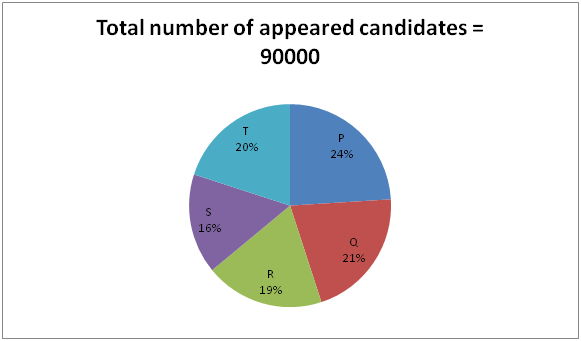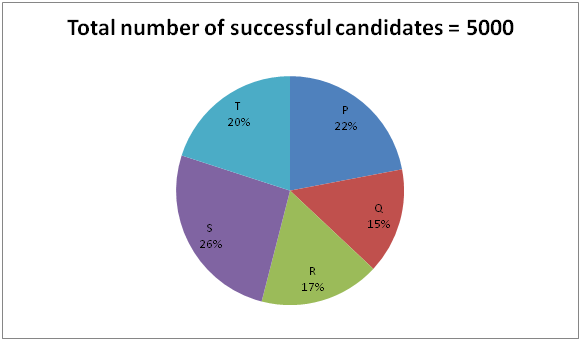# NIACL AO Mains– Quantitative Aptitude Questions Day- 10

Dear Readers, Bank Exam Race for the Year 2019 is already started, To enrich your preparation here we have providing new series of Practice Questions on Quantitative Aptitude – Section. Candidates those who are preparing for NIACL AO Mains 2019 Exams can practice these questions daily and make your preparation effective.

[WpProQuiz 5149]

Directions (Q. 1 – 5): In the following questions, two equations I and II are given. You have to solve both the equations and give answer as,

a) If x > y

b) If x ≥ y

c) If x < y

d) If x ≤ y

e) If x = y or the relation cannot be established

1)

I) 2x2 + 21x + 49 = 0

II) 4y2 + 48y + 23 = 0

2)

I) 5x – 3y = -1

II) 3x – 2y = 0

3)

I) x2 – 24x – 81 = 0

II) y2 – 21y – 72 = 0

4)

I) x = (∛157464 ÷ 6) + (72 × 56) ÷ 64

II) y = ∜20736 + 82 + (512 ÷ 128)

5)

I) 3x2 + 25x + 52 = 0

II) 2y2 + 23y + 66 = 0

Directions (Q. 6 – 10): Study the following information carefully and answer the given questions.

The following pie chart 1 shows the total number of students who appeared for IAS exam from 5 different states and the pie chart 2 shows the total number of successful candidates of IAS examination from 5 different states.6) Total number of successful candidates of IAS examination from state S is approximately what percentage of total number of candidates appeared for IAS examination from state P?

a) 12 %

b) 25 %

c) 34 %

d) 6 %

e) 18 %

7) Find the ratio between the total number of candidates appeared for IAS examination from state Q and S together to that of total number of successful candidates of IAS examination from state P, Q, R and T together?

a) 9 : 1

b) 111: 23

c) 75: 28

d) 52: 13

e) None of these

8) Find the difference between the total number of unsuccessful candidates of State R to that of State S?

a) 2780

b) 3360

c) 3150

d) 3025

e) None of these

9) Find the average number of unsuccessful candidates of State P, Q and R together?

a) 17500

b) 18300

c) 17900

d) 18700

e) None of these

10) Total number of candidates appeared for IAS examination from state P and Q together is what percentage more/less than the total number of candidates appeared for IAS examination from S and T together?

a) 15 %

b) 20 %

c) 30 %

d) 25 %

e) None of these

Direction (1-5 ) :

I) 2x2 + 21x + 49 = 0

2x2 + 14x + 7x + 49 = 0

2x (x + 7) + 7(x + 7) = 0

(2x + 7) (x + 7) = 0

X = -7/2, -7 = -3.5, -7

II) 4y2 + 48y + 23 = 0

4y2 + 2y + 46y + 23 = 0

2y (2y + 1) + 23 (2y + 1) = 0

(2y + 23) (2y + 1) = 0

Y = -23/2, -1/2 = -11.5, -0.5

Can’t be determined

5x – 3y = -1 —> (1)

3x – 2y = 0 —> (2)

By solving (1) and (2), we get,

X = -2, y = -3

X > y

I) x2 – 24x – 81 = 0

(x – 27) (x + 3) = 0

X = 27, -3

II) y2 – 21y – 72 = 0

(y – 24) (y + 3) = 0

Y = 24, -3

Can’t be determined

I) x = (∛157464 ÷ 6) + (72 × 56) ÷ 64

X = (54/6) + (72*56)/64

X = 9 + 63 = 72

II) y = ∜20736 + 82 + (512 ÷ 128)

Y = 12 + 64 + 4 = 80

X < y

I) 3x2 + 25x + 52 = 0

3x2 + 12x + 13x + 52 = 0

3x (x + 4) + 13(x + 4) = 0

(3x + 13) (x + 4) = 0

X = -13/3, -4 = -4.33, -4

II) 2y2 + 23y + 66 = 0

2y2 + 12y + 11y + 66 = 0

2y (y + 6) + 11(y + 6) = 0

(2y + 11) (y + 6) = 0

Y = -11/2, -6 = -5.5, -6

X > y

Direction (6-10) :

Total number of successful candidates of IAS examination from state S

= > 5000*(26/100) = 1300

Total number of candidates appeared for IAS examination from state P

= > 90000*(24/100) = 21600

Required % = (1300/21600)*100 = 6 %

The total number of candidates appeared for IAS examination from state Q and S together

= > [(21 + 16)/100]*90000 = 33300

The total number of successful candidates of IAS examination from state P, Q, R and T together

= > [(22 + 15 + 17 + 20)/100]*5000 = 3700

Required ratio = 33300: 3700 = 333: 37 = 9 : 1

The total number of unsuccessful candidates of State R

= > [90000*(19/100)] – [5000*(17/100)]

= > 17100 – 850 = 16250

The total number of unsuccessful candidates of State S

= > [90000*(16/100)] – [5000*(26/100)]

= > 14400 – 1300 = 13100

Required difference = 16250 – 13100 = 3150

The total number of unsuccessful candidates of State P, Q and R together

= > {[90000*(24/100)] – [5000*(22/100)]} + {[90000*(21/100)] – [5000*(15/100)]} + {[90000*(19/100)] – [5000*(17/100)]}

= > (21600 – 1100) + (18900 – 750) + (17100 – 850)

= > 20500 + 18150 + 16250

= > 54900

Required average = 54900/3 = 18300